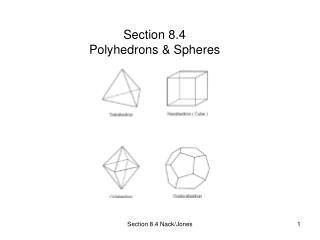DownloadDownload PresentationSection 8.4 Polyhedrons & Spheres

# Section 8.4 Polyhedrons & Spheres

Download Presentation## Section 8.4 Polyhedrons & Spheres

- - - - - - - - - - - - - - - - - - - - - - - - - - - E N D - - - - - - - - - - - - - - - - - - - - - - - - - - -
##### Presentation Transcript

1. Section 8.4Polyhedrons & Spheres Section 8.4 Nack/Jones

2. Polyhedron • Plural: polyhedrons or polyhedra • A solid bounded by plane regions. • The faces of the polyhedrons are polygons • The edges are the line segments common to these polygons • Vertices are the endpoints of the edges • Convex: Each face determines a plane for which all remaining faces lie on the same side of the plane. p.420. • Concave: Two vertices and the line segment containing them lies in the exterior of the polyhedron. Section 8.4 Nack/Jones

3. Euler’s Equation • Theorem 8.4.1: The number of vertices V, the number of edges, E, and the number of faces F of a polyhedron are related by the equation. V + F = E + 2 Where V = # of vertices F = # of faces E = # of edges Example 1 p. 420 Section 8.4 Nack/Jones

4. Regular Polyhedron • A regular polyhedron is a convex polyhedron whose faces are congruent regular polygons arranged in such a way that adjacent faces form congruent dihedral angles (the angle formed when two edges intersect). Section 8.4 Nack/Jones

5. Spheres • Three Characteristics • A sphere is the set of all points at a fixed distance r from a given point O. Point O is known as the center of the sphere. • A sphere is the surface determined when a circle (or semicircle) is rotated about any of its diameters. • A sphere is the surface that represents the theoretical limit of an “inscribed” regular polyhedron whose number of faces increase without limit. Section 8.4 Nack/Jones

6. Surface Area and Volume of a Sphere • Theorem 8.4.2: The surface area S of a sphere whose radius has length r is given by S = 4r² • Theorem 8.4.3: The volume V of a sphere with radius of length r is given by V =4/3 r3 Example 4 – 6 p. 424 • Solids of Revolution: • Revolving a semi circle = sphere • Revolving circle around line = torus p. 425 - 426 Section 8.4 Nack/Jones